# Series and Parallel

When we use discrete components in circuits either we connect in series or parallel to the Power supply. This article describes about Series Resistor, Parallel Resistor, and Series Parallel Capacitor connections.

By using simple Calculations we can find the total Resistance and Capacitance Value in circuit.

Series Circuit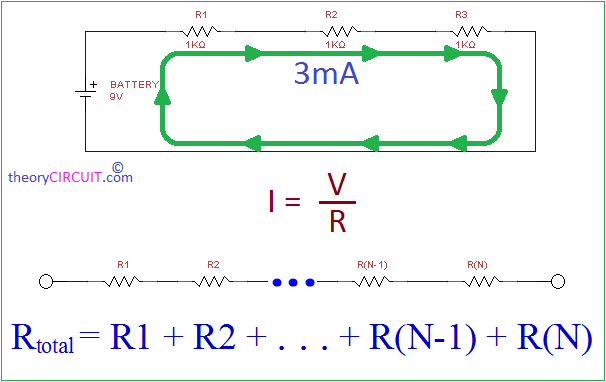Three 1KΩ Resistors are Connected series and Powered by 9V battery. When resistors in series, the current flow in each resistor is same 3mA.To caculate Total Resistance add each resistance Value cumulatively. Three 1KΩ Resistors R1, R2, R3 connected in series hence the total resistance is 1K+1K+1K = 3KΩ.

Parallel Circuit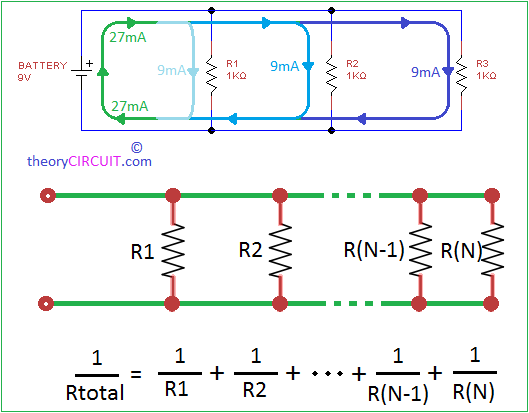Three 1KΩ Resistors are connected in Parallel and powered by 9V battery. The current flow varies in each node depends on the Resistor Value. Here total current is 27mA and the current flow through R1 is 9mA, R2 is 9mA and R3 is 9mA. When the Resistor Value gets change the current flow value also gets change.

Total resistance value can be Obtained by formula stated in diagram.

Equal Resistors in Parallel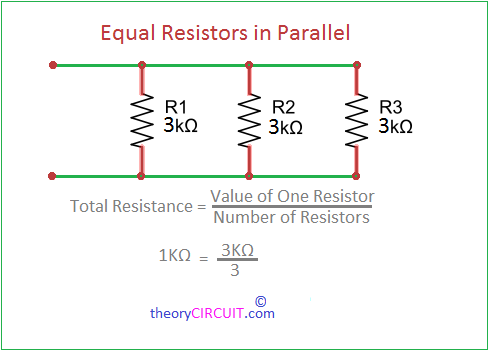When same Value resistors connected in parallel then you can calculate total Resistance value by divide value of one resistor by number of resistors. The circuit contains three 3KΩ Resistors by dividing 3K by 3 gives 1KΩ Resistor value.

## Measuring Resistors in Series and Parallel Connection

Bring the calculations into experiment to do this you need a bread board, bunch of Resistors and Multimeter enabled with Ω measurement.

Connect the resistors on bread board in different way as shown in pictures and take multimeter and measure the resistance value, by the way check the theoretical value by using corresponding series, parallel formula.

You may get some little difference that is can be taken as tolerance value. Because each resistor will have some tolerance value.

### Series Capacitors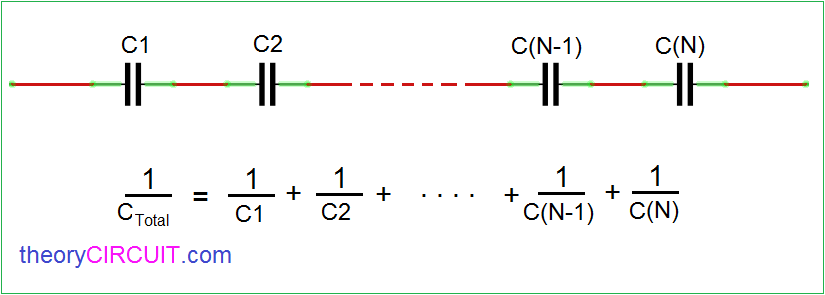Connecting Capacitors in Series makes the reduction in total Capacitance value, you can calculate the total capacitance value of series circuit capacitors by using formula stated in picture. You may ask why reduction happens to the capacitance in series connection, Answer for your question is here.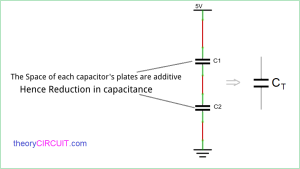The space of each capacitors plates are additive hence Value Reduced in total capacitance.

### Parallel Capacitor CircuitWhen Connecting capacitors in parallel connection each value added together and the total capacitance value gets increase.

How Parallel Capacitor value Increases?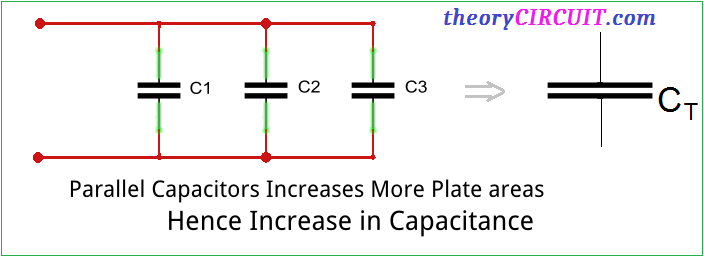Parallel Capacitors increases more plate areas hence increase in Capacitance value.